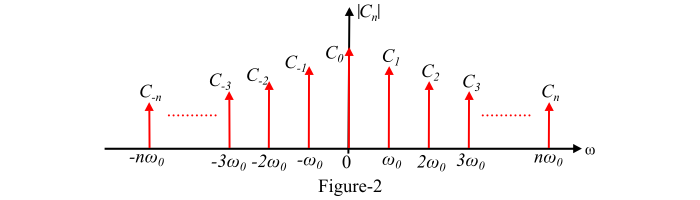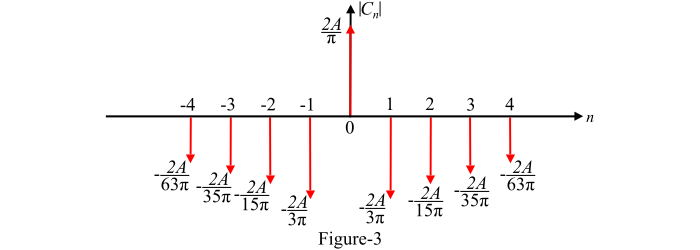# What is Fourier Spectrum? – Theory and Example

The graph plotted between the Fourier coefficients of a periodic function $x(t)$ and the frequency (ω) is known as the Fourier spectrum of a periodic signal.

The Fourier spectrum of a periodic function has two parts −

• Amplitude Spectrum − The amplitude spectrum of the periodic signal is defined as the plot of amplitude of Fourier coefficients versus frequency.

• Phase Spectrum − – The plot of the phase of Fourier coefficients versus frequency is called the phase spectrum of the signal.

The amplitude spectrum and phase spectrum together are known as Fourier frequency spectra of the periodic signal $x(t)$. This type of representation of a periodic signal is known as frequency domain representation.

The Fourier frequency spectra exists only at discrete frequencies, i.e., at , where, n = 0, 1, 2, 3,… . Therefore, the Fourier frequency spectra is also known as discrete spectra or line spectra. The envelope of the Fourier frequency spectra depends only upon shape of the pulse, but not upon the period of repetition.

The trigonometric Fourier series representation of a periodic function $x(t)$ contains both sine and cosine terms with positive and negative amplitude coefficients $a_{n}$ and $b_{n}$but does not have phase angles.

## Single-Sided Spectra

The cosine Fourier series representation of a periodic signal x(t) has only positive amplitude coefficients $A_{n}$ with phase angle $\theta_{n}$. Hence, we can plot amplitude spectrum ($A_{n}$ versus $\omega$) and the phase spectrum ($\theta_{n}$ versus $\omega$ ).

In the cosine representation, the Fourier coefficients exist only for positive frequencies. Therefore, this spectra is called the single-sided spectra.

Figure-1 represents the spectrum of a trigonometric (cosine) Fourier series extending from 0 to ∞, producing a one sided spectrum because no negative frequencies exist in this representation.## Two-Sided Spectra

The exponential Fourier series representation of a periodic function $x(t)$ has amplitude coefficients $C_{n}$ which are complex and can be represented by magnitude and phase. Hence, we can plot the amplitude spectrum ($|C_{n}|$ versus $\omega$) and the phase spectrum ($\angle C_{n}\:versus\:\omega$).

As in the exponential representation, the spectra can be plotted for both positive and negative frequencies. Therefore, this spectra is known as two-sided spectra.

Figure-2 represents the spectrum of a complex exponential Fourier series extending from (−∞ to ∞),producing a two-sided spectrum.Also, if $C_{n}$ is complex number, then

$$\mathrm{C_{n}=|C_{n}|e^{j\theta_{n}}}$$

$$\mathrm{C_{-n}=|C_{n}|e^{-j\theta_{n}}}$$

And

$$\mathrm{C_{n}=|C_{-n}|}$$

Therefore, the amplitude spectrum of the exponential Fourier series is symmetrical about the vertical axis passing through the origin, i.e., the magnitude spectrum exhibits even symmetry and the phase spectrum is antisymmetrical about the vertical axis passing through the origin, i.e., the phase spectrum exhibits odd symmetry.

Also, when the periodic signal $x(t)$ is real, then

$$\mathrm{C_{-n}=C_{n}^{*}}$$

i.e.$C_{-n}$ is the complex conjugate of the exponential coefficient $C_{n}$.

## Numerical Example

The exponential Fourier series of a periodic function is given by,

$$\mathrm{x(t)=\sum_{n=−\infty}^{\infty}\frac{2A}{\pi(1-4n^{2})}e^{j2nt}=\frac{2A}{\pi}+\frac{2A}{\pi}\sum_{\substack{n=-\infty\ n eq 0}}^{\infty}\left ( \frac{e^{j2nt}}{1-4n^{2}} \right ) }$$

Plot the frequency spectrum for the given function.

Solution

The given exponential Fourier series is,

$$\mathrm{x(t)=\sum_{n=−\infty}^{\infty}\frac{2A}{\pi(1-4n^{2})}e^{j2nt}=\frac{2A}{\pi}+\frac{2A}{\pi}\sum_{\substack{n=-\infty\ n eq 0}}^{\infty}\left ( \frac{e^{j2nt}}{1-4n^{2}} \right )}$$

Here, the exponential Fourier coefficients are −

$$\mathrm{C_{0}=\frac{2A}{\pi}\:\:and\:\:C_{n}=\frac{2A}{\pi(1-4n^{2})}}$$

Therefore,

$$\mathrm{C_{1}=C_{-1}=\frac{2A}{\pi[1-4(1)^{2}]}=-\frac{2A}{3\pi}}$$

$$\mathrm{C_{2}=C_{-2}=\frac{2A}{\pi[1-4(2)^{2}]}=-\frac{2A}{15\pi}}$$

$$\mathrm{C_{3}=C_{-3}=\frac{2A}{\pi[1-4(3)^{2}]}=-\frac{2A}{35\pi}}$$

$$\mathrm{C_{4}=C_{-4}=\frac{2A}{\pi[1-4(4)^{2}]}=-\frac{2A}{63\pi}}$$

And so on…

Hence, the frequency spectrum of the given function can be plotted as shown in Figure-3.